# Robotics Laboratory

## Curvature-Based Computation of Antipodal Grasps

It is well known that antipodal grasps can be achieved on curved objects in the presence of friction. For example, in the closed cubic spline to the left, two fingers placed at any of the eight pairs of points (numbered the same) with opposite but collinear normals form a force-closure grasp. Each pair of points are referred to as antipodal points .

I have designed an efficient algorithm that finds, up to numerical resolution, all pairs of antipodal points on a closed, simple, and twice continuously differentiable planar curve. Dissecting the curve into segments everywhere convex or everywhere concave, the algorithm performs simultaneous marching on a pair of such segments with provable convergence and interleaves marching with numerical bisection. The algorithm makes use of new insights into the differential geometry at a pair of antipodal points.

Curve dissection relies on simple inflection points where the curvature is zero. The preprocessing stage also includes an algorithm with quadratic convergence that constructs common tangent lines of two curve segment.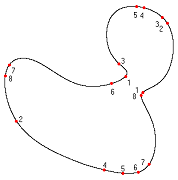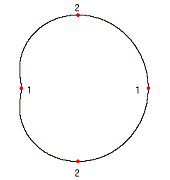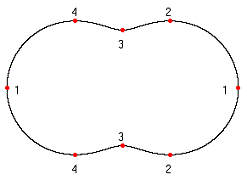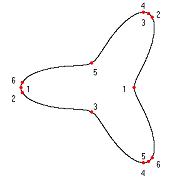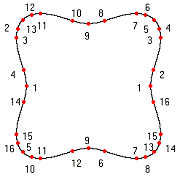The above shows antipodal points found on a limacon ( r = 4 + 2.5 cos(phi) in polar coordinates), an elliptic lemniscate (r = sqrt(36 cos^2(phi) + 9 sin^2(phi))), and two curves with convexities that have equations r = 3/(1+0.5 cos (3*phi)) and r = 4.5/(1+0.2 cos (4*phi)), respectively.

Let n be the number of inflection points and m the number of pairs of antipodal points. There are O(n^2) pairs of segments after the preprocessing. The total number of calls to the marching and bisection procedures is O(n^2 + m). In experimental trials, the number of iteration steps taken by any numerical procedures was at most 20 and the number of iteration steps by any primitives employed by a procedure was at most 15.

Computation involving curves used to sacrifice completeness and efficiency due to the nonlinear nature of the curves. In this problem, a conventional nonlinear programming approach, inherently local, would be slow and not guarantee to always find antipodal points, not to mention all of them. Curve dissection and the combination of marching with bisection have proven to be effective (and numerically complete) for processing and optimization.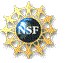This material is based upon work supported by an

NSF

Any opinions, findings, and conclusions or recommendations expressed in this material are those of the author(s) and do not necessarily reflect the views of the National Science Foundation.